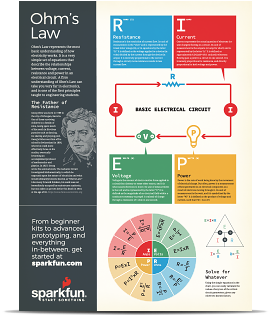# Voltage, Current, Resistance, and Ohm's Law

Pages

## Electricity Basics

When beginning to explore the world of electricity and electronics, it is vital to start by understanding the basics of voltage, current, and resistance. These are the three basic building blocks required to manipulate and utilize electricity. At first, these concepts can be difficult to understand because we cannot "see" them. One cannot see with the naked eye the energy flowing through a wire or the voltage of a battery sitting on a table. Even the lightning in the sky, while visible, is not truly the energy exchange happening from the clouds to the earth, but a reaction in the air to the energy passing through it. In order to detect this energy transfer, we must use measurement tools such as multimeters, spectrum analyzers, and oscilloscopes to visualize what is happening with the charge in a system. Fear not, however, this tutorial will give you the basic understanding of voltage, current, and resistance and how the three relate to each other.

Georg Ohm

### Covered in this Tutorial

• How electrical charge relates to voltage, current, and resistance.
• What voltage, current, and resistance are.
• What Ohm's Law is and how to use it to understand electricity.
• A simple experiment to demonstrate these concepts.